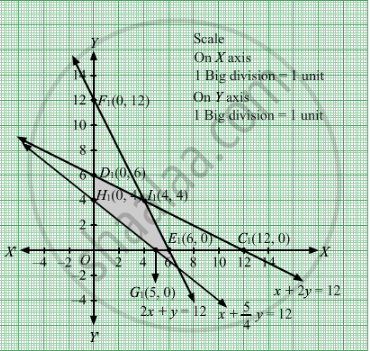# A Manufacturer Has Three Machines Installed in His Factory. Machines I and Ii Are Capable of Being Operated for at Most 12 Hours Whereas Machine Iii Must Operate at Least for 5 Hours a Day - Mathematics

Sum

A manufacturer has three machines installed in his factory. machines I and II are capable of being operated for at most 12 hours whereas Machine III must operate at least for 5 hours a day. He produces only two items, each requiring the use of three machines. The number of hours required for producing one unit each of the items on the three machines is given in the following table:

 Item Number of hours required by the machine AB I II III 12 21 15/4

He makes a profit of Rs 6.00 on item A and Rs 4.00 on item B. Assuming that he can sell all that he produces, how many of each item should he produces so as to maximize his profit? Determine his maximum profit. Formulate this LPP mathematically and then solve it.

#### Solution

Let  units of item A and units of item B be manufactured.
Therefore, $x, y \geq 0$

As we are given,

 Item Number of hours required by the machine AB I II III 12 21 15/4

Machines I and II are capable of being operated for at most 12 hours whereas Machine III must operate at least for 5 hours a day.
According to question, the constraints are

$x + 2y \leq 12$

$2x + y \leq 12$

$x + \frac{5}{4}y \geq 5$

He makes a profit of Rs 6.00 on item A and Rs 4.00 on item B.
Profit made by him in producing x items of A and y items of B is 6x + 4y.
Total profit Z = $6x + 4y$ which is to be maximised
Thus, the mathematical formulat​ion of the given linear programmimg problem is
Max Z =$6x + 4y$ subject to

$x + 2y \leq 12$
$2x + y \leq 12$
$x + \frac{5}{4}y \geq 5$

$x, y \geq 0$

First we will convert inequations into equations as follows :
x + 2y = 12, 2x + y = 12,

$x + \frac{5}{4}y = 5$ , x = 0 and y = 0

Region represented by x + 2y ≤ 12:
The line x + 2y = 12 meets the coordinate axes at A1(12, 0) and B1(0, 6) respectively. By joining these points we obtain the line x + 2y = 12.Clearly (0,0) satisfies the x + 2y = 12. So, the region which contains the origin represents the solution set of the inequation x + 2y ≤ 12.
Region represented by 2x + y ≤ 12:
The line 2x + y = 12 meets the coordinate axes at C1(6, 0) and D1(0, 12) respectively. By joining these points we obtain the line 2x + y = 12. Clearly (0,0) satisfies the inequation 2x + y ≤ 12. So,the region which contains the origin represents the solution set of the inequation 2x + y ≤ 12.
Region represented by $x + \frac{5}{4}y = 5$
The line $x + \frac{5}{4}y = 5$ meets the coordinate axes at E1(5, 0) and F1(0, 4) respectively. By joining these points we obtain the line
$x + \frac{5}{4}y = 5$.  Clearly (0,0) does not satisfies the inequation
$x + \frac{5}{4}y \geq 5$  So , the Region represented by x ≥ 0 and y ≥ 0:
Since, every point in the first quadrant satisfies these inequations. So,the region which does not contains the origin represents the solution set of the inequation $x + \frac{5}{4}y \geq 5$
region represented by the inequations x ≥ 0, and ≥ 0.
The feasible region determined by the system of constraints x + 2y ≤ 12, 2x + y ≤ 12,
$x + \frac{5}{4}y \geq 5$ , x ≥ 0, and y ≥ 0 are as follows.The corner points are B1(0, 6), G1(4, 4), C1(6, 0), E1(5, 0) and F1(0, 4).

The values of Z at these corner points are as follows

 Corner point Z = 6x + 4y B1 24 G1 40 C1 36 E1 30 F1 16

The maximum value of Z is 40 which is attained at G1(4, 4).

Thus, the maximum profit is Rs 40 obtained when 4 units each of item A and B are manufactured.
Concept: Graphical Method of Solving Linear Programming Problems
Is there an error in this question or solution?

#### APPEARS IN

RD Sharma Class 12 Maths
Chapter 30 Linear programming
Exercise 30.4 | Q 2 | Page 50